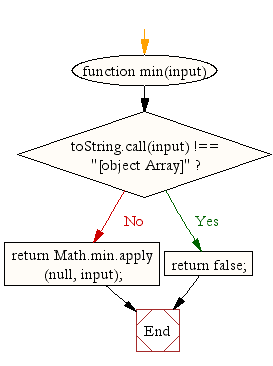# JavaScript: Find the lowest value in an array

## JavaScript Math: Exercise-7 with Solution

Write a JavaScript function to find the lowest value in an array.

Test Data :
console.log(min([12,34,56,1]));
console.log(min([-12,-34,0,-56,-1]));
1
-56

Pictorial Presentation:Sample Solution:-

HTML Code:

``````<!DOCTYPE html>
<html>
<meta charset="utf-8">
<title>Javascript random function</title>
<body>

</body>
</html>
```
```

JavaScript Code:

``````function min(input) {
if (toString.call(input) !== "[object Array]")
return false;
return Math.min.apply(null, input);
}

console.log(min([12,34,56,1]));
console.log(min([-12,-34,0,-56,-1]));
```
```

Sample Output:

```1
-56
```

Flowchart:Live Demo:

See the Pen javascript-math-exercise-7 by w3resource (@w3resource) on CodePen.

Improve this sample solution and post your code through Disqus

What is the difficulty level of this exercise?

Test your Programming skills with w3resource's quiz.

﻿

## JavaScript: Tips of the Day

Returns an array of n-tuples of consecutive elements

Example:

```const tips_arr = (n, arr) =>  n > arr.length ? [] : arr.slice(n - 1).map((v, i) =>[...arr.slice(i, i + n - 1), v]);
console.log(tips_arr(2, [1, 2, 3, 4, 5]));
console.log(tips_arr(3, [1, 2, 3, 4, 5]));
console.log(tips_arr(5, [1, 2, 3, 4]));
```

Output:

```[[1, 2], [2, 3], [3, 4], [4, 5]]
[[1, 2, 3], [2, 3, 4], [3, 4, 5]]
[]
```